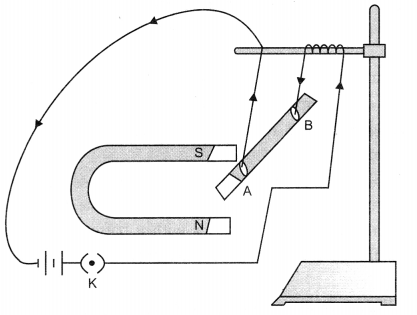# Magnetic Effects of Electric Current Class 10 Important Questions Science Chapter 13

Solved the very best collection of Magnetic Effects of Electric Current Class 10 Science Important Questions and Answers Chapter 13 Pdf from the latest NCERT edition books, It will help you in scoring more marks in CBSE Exams.

## Magnetic Effects of Electric Current Class 10 Important Questions with Answers Science Chapter 13

### Magnetic Effects of Electric Current Class 10 Important Questions Very Short Answer Type

Question 1.
When the total current drawn by all the appliances at a particular time exceeds the bearing capacity of that wire, the wires of the domestic wiring gets heated this is called overloading.

Question 2.
Name the type of current:
(a) used in household supply,
(b) given by a cell.   (CBSE 2012)
(a) Alternating current
(b) Direct current.

Question 3.
State the direction of magnetic field in the following case.   (CBSE 2012)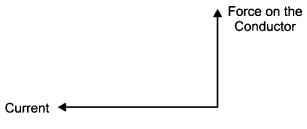Perpendicular to the plane of paper in the outward direction by using Fleming’s left hand rule.

Question 4.
Give one application of electromagnetic induction.   (CBSE 2012)
This phenomenon is used in electric generator.

Question 5.
Name the physical quantities which are indicated by the direction of thumb and forefinger in the Fleming’s right hand rule?   (CBSE 2012)
In Fleming’s right hand rule, thumb indicates – direction of motion of the conductor;
forefinger indicates – direction of magnetic field.

Question 6.
A positively charged particle (alpha-particle) projected towards west is deflected towards north by a magnetic field. The direction of magnetic field is
(a) towards north
(b) towards east
(c) downward
(d) upward   (CBSE 2012)
(d) Upward.

### Magnetic Effects of Electric Current Class 10 Important Questions Short Answer Type

Question 1.
Why is a series arrangement not used for connecting domestic electrical appliances in a circuit?  (AI 2008)
In order to provide equal potential difference to each appliance they are not connected in series.

Question 2.
What is meant by the term magnetic field? Why does a compass needle show deflection when brought near a bar magnet?   (AI 2008)
The region or space around a magnet where magnetic force can be experienced by other magnetic substance is called magnetic field. It is measured in tesla or weber/m2. The compass needle show deflection due to the effect of magnetic field . around the bar magnet.

Question 3.
Draw a diagram to show the magnetic field around a current carrying solenoid. What do you interpret from the pattern of the field lines?   (AI 2008)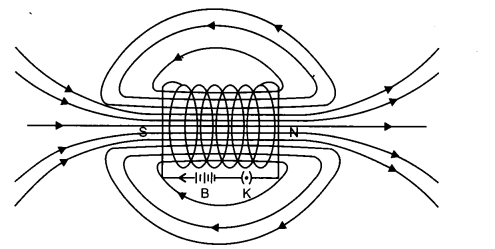We can interpret that these lines

• do not intersect
• form closed loops

Question 4.
How is an electromagnet different from a permanent magnet?   (Foreign 2008)
Electromagnet retains magnetism as long as the current flows in the enveloping coil, while a permanent magnet retains its magnetism all the time.

Question 5.
An electron enters a magnetic field at a right angle to the field direction.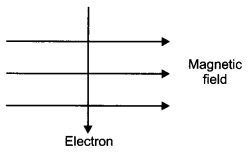(a) State the rule to find the direction of force
(b) What will be direction of force acting on the electron in the above case? (Foreign 2008)
(a) Fleming’s Left Hand Rule: Stretch the first three fingers of the left hand mutually perpendicular to each other such that the fore-fingers points the direction of magnetic field, the middle finger points the direction of current, then the thumb will indicate the direction of force experienced by the conductor. It is to be applied when the current and field are perpendicular to each other.(b) Vertically downward or into the plane of the paper.

Question 6.
Draw the pattern of magnetic field lines around a straight conductor carrying electric current. Apply right-hand thumb rule to mark the direction of magnetic field.   (CBSE 2007C)
Magnetic field around a straight current carrying conductor: Insert a wire carrying current through a hole at the middle of a cardboard when a current I is passed through the wire. Surrounding the wire magnetic field will be created when you sprinkle iron filings, on the board they will all settle in concentric rings. The direction of north pole is given by the arrow. Reversal of current will reverse the magnetic field.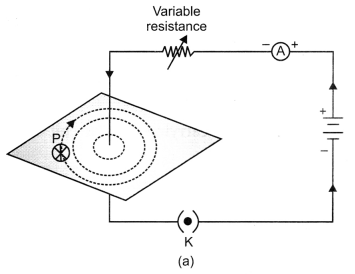Fig. (a) A pattern of concentric circles indicating the field lines of a magnetic field around a straight conducting wire. The arrows in the circles show the direction of the field lines.(b) A dose up of the pattern obtained.

Question 7.
Draw the pattern of magnetic field lines in and around a long current carrying solenoid indicate
(i) the polarity at each end and
(ii) the direction of magnetic field inside the solenoid.   (CBSE 2008C)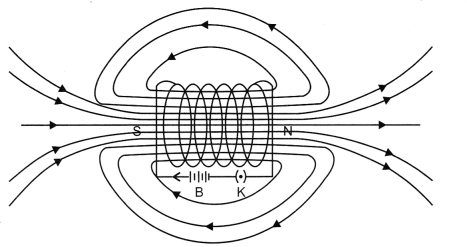Question 8.
State and explain how right hand thumb rule indicates magnetic field.   (CBSE 2011)
Right hand thumb rule-Imagine holding the current carrying straight conductor in your right hand such that the thumb points towards the direction of current. Then the fingers of right hand wrap around the conductor and shows the direction of the magnetic field formed around it.Question 9.
What is an electric fuse? What material is selected for fuse wire?  (CBSE 2011)
An electric fuse is a device which is used and connected in series of an electric circuit as a safety device to prevent the damage caused by short circuiting or overloading of the circuit.

Fuse wire is made up of small thin wire whose melting point is low. So that it can control the amount of current flowing through it, if an extra current passes through it then the fuse wire melts and breaks the circuit thereby preventing the damage of electric appliances. An alloy of Pb + Sn is used for the same.

Question 10.
How will the magnetic field produced in a current carrying circular coil change if we:
(i) increase the value of current?
(ii) increase the distance from the coil?
(in) increase the number of turns of coil?  (CBSE 2011)
(i) If the value of current increases, the magnetic field produced in a current carrying circular coil will also increase.
(ii) The magnetic field decreases if the distance from the coil increases.
(iii) Magnetic field increases if there is increase in the number of turns of coil.

Question 11.
Insulation cover of which colour is conventionally used for earth wire? Why is an earth wire connected to metallic parts of appliances?   (CBSE 2011)
The earth wire is of green colour or may be yellow in some cases.
The earth wire provides a low resistance conducting path for electric current. If there is any leakage of current then the user would not get any current because the current flows down into the earth and keeps the potential of the appliance and earth same.

Question 12.
Identify the poles of the magnet in the given figure (i) and (ii).   (CBSE 2011)Field lines emerge from north pole (N) and merge at the south pole (S) as shown in both the figuresQuestion 13.
Explain the role of fuse in series with any electrical appliance in an electric circuit. Why should a fuse with defined rating for an electric circuit not be replaced by one with a larger rating? (CBSE 2011)
An electric fuse is a device which is used and connected in series of an electric circuit as a safety device to prevent the damage caused by short circuiting or overloading of the circuit.

Fuse wire is made up of small thin wire whose melting point is low. So that it can control the amount of current flowing through it, if an extra current passes through it then the fuse wire melts and breaks the circuit thereby preventing the damage of electric appliances. An alloy of Pb + Sn is used for the same.

Electric circuit cannot be replaced by one with a larger rating as fuse wire will not melt even on high (exceeding permitted value) current, which causes excessive heat and ultimately catches fire.

Question 14.
The magnetic field associated with a current-carrying straight conductor is in anticlockwise direction. If the conductor was held along the east-west direction, what will be the direction of current through it? Name and state the rule applied to determine the direction of current.  (CBSE 2011)
Right hand thumb rule-Imagine holding the current carrying straight conductor in your right hand such that the thumb points towards the direction of current. Then the fingers of right hand wrap around the conductor and shows the direction of the magnetic field formed around it.The direction of the current would be east-west, this can be determined by the right hand thumb rule.

Question 15.
Two circular coils A and B are placed close to each other. If the current in the coil A is changed, will some current be induced in the coil B? Give reason.   (CBSE 2011)
Yes, when a current in coil A changes, magnetic field lines linked with coil B also change. Hence, due to change in number of magnetic field lines, there is an induced current in coil B.

Question 16.
How will the magnetic field produced at a point due to a current-carrying circular coil change if we:
(i) increase the current flowing through the coil?
(ii) reverse direction of current through the coil?
(iii) increases the number of turns in the coil?   (CBSE 2011)
(i) If the value of current increases, the magnetic field produced in a current carrying circular coil will also increase.
(ii) The magnetic field direction will be reversed.
(iii) Magnetic field increases if there is increase in the number of turns of coil.

Question 17.
(a) Mention the factors on which the direction of force experienced by a current – carrying conductor placed in a magnetic field depend.
(b) Under what condition is the force experienced by a current-carrying conductor placed in a magnetic field maximum?
(c) A proton beam is moving along the direction of a magnetic field. What force is
acting on proton beam?   (CBSE 2011)
(a) The direction of force experienced by the current-carrying conductor depends on:
(i) direction of current and
(ii) direction of magnetic field.

(b) When the direction of current is at right angle to the direction of magnetic field, the force is maximum.

(c) No force is experienced by the proton beam. As proton beam is moving along the direction of magnetic field.

Question 18.
A coil of insulated copper wire is connected to a galvanometer. What will happen if a bar magnet is:
(a) pushed into the coil,
(b) withdrawn from inside the coil,
(c) held stationary inside the coil?   (CBSE 2011)
(a) If a bar magnet is pushed in the coil, the galvanometer will show deflection as the electric current is generated.

(b) When a bar magnet is withdrawn from the coil the deflection in the galvanometer will be in the opposite direction. This is because the direction of the induced current changes.

(c) When a bar magnet is held stationary in the coil, there will be no deflection. There is no current induced when the bar magnet is stationary as there is no charge in the magnetic field lines linked with the coil.

Question 19.
State the rule to determine the direction of a
(a) magnetic field produced around a straight conductor carrying current.
(b) force experienced by a current-carrying straight conductor placed in a magnetic
field which is perpendicular to it. (CBSE 2011)
(a) Direction of magnetic field produced around a straight current-carrying conductor is given by right-hand thumb rule. If we hold a current-carrying conductor in a right hand in such a way that the stretched thumb is along the direction of current, then the curly fingers around the conductor represent the direction of magnetic field lines.

(b) Direction of force experienced by a current-carrying straight conductor placed in a magnetic field which is perpendicular to it, is given by Fleming’s left hand rule.

Stretch the thumb, forefinger and middle finger of left hand in such a way that they are mutually perpendicular to each other. If the forefinger points to the direction of magnetic field and the middle finger points to the direction of current, then the thumb will point to the direction of motion or the force acting on the conductor.

Question 20.
The given magnet is divided into three parts A, B and C
Name the parts where the strength of the magnetic field is :
(i) maximum
(ii) minimum. How will the density of magnetic field lines differ at these parts?  (CBSE 2012)
If the magnet is kept intact, then the maximum strength will be at poles i.e. is at A and C. The minimum strength will be at C.

The density will be more at the poles than at the centre near C, the magnetic field lines are crowded at the poles and they spread away at C.

If the bar magnet is divided into three pieces and kept away from each other than each piece will behave like a magnet and all three will show same magnetic strength.

Question 21.
A compass needle is placed near a current-carrying wire. State your observation for the following cases, and give reason for the same in each case.
(a) Magnitude of electric current in the wire is increased.
(b) The compass needle is displaced away from the wire.   (CBSE 2012)
(a) Observation: The deflection of the needle increases.
Reason: Magnetic field strength due to current-carrying wire increases as current in the wire increases.

(b) Observation: The deflection in the compass needle decreases as its displacement from the current-carrying wire increases.
Reason: The strength of magnetic field reduces with the increase in distance from the wire.

Question 22.
(a) Two magnets are lying side by side as shown below. Draw magnetic field line between poles P and Q.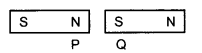(b) What does the degree of closeness of magnetic field lines near the poles signify?   (CBSE 2012)
(a)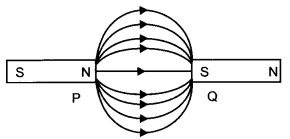(b) The degree of closeness of magnetic field lines near the poles signify that the field is stronger there.

Question 23.
Magnetic field lines of two magnets are shown in Fig. A and Fig. B.Select the figure that represents the correct pattern of field lines. Give reasons for your answer. Also name the poles of the magnets facing each other.   (CBSE 2012)
Figure B represents the correct pattern of field lines. The two poles facing each other are same may be N-N pole or S-S as the field lines are showing repulsion by moving away from each other.

In Fig. A the filed lines are crossing each other and the intersection is seen. But in Fig. B the field lines are parallel to each other and do not intersect.

Question 24.
What is short circuiting? State one factor/condition that can lead to it. Name a device in the household that acts as a safety measure for it. State the principle of its working.   (CBSE 2012)
Short circuiting: When the non-insulated live wire comes in contact with the earth wire or the neutral wire the spark is produced resulting into fire is called short circuiting.

Factor: When the insulation of the wire is worn out or melts.
Safety device is Fuse.

Principle for its working: It works on joule’s Law of heating effect caused due to electric current.
H = I2RT
As per this law the heat produced by the electric current is directly proportional to :

1. The square of current flowing through the wire here it is a fuse wire.
2. The resistance of the wire used in fuse
3. The time for which the current is flowing through the wire.

If the current flowing through the fuse wire increases, then the wire melts due to its lower melting point thereby protecting the electric appliances from any damage.

Question 25.
Write one application of each of the following:
(a) Right-hand thumb rule
(b) Fleming’s left hand rule
(c) Fleming’s right hand rule.   (CBSE 2012)
(a) Right-hand thumb rule is used to find the direction of magnetic field in a coil of wire and the electric current in a straight conductor.

(b) Fleming’s left hand rule is used to find the direction of force exerted on a current¬carrying conductor placed in a magnetic field as in electric motor.

(c) Fleming’s right hand rule is used to find the direction of induced current in a closed circuit placed in changing magnetic field as in electric generator.

Question 26.
(a) Mention the effect of electric current on which the working of an electrical fuse is based.
(b) Draw a schematic labelled diagram of a domestic circuit which has a provision of
a main fuse, meter, one light bulb and a socket.
(a) The working of electric fuse is based on the heating effect of electric current.
(b) An electric fuse is a device which is used and connected in series of an electric circuit as a safety device to prevent the damage caused by short circuiting or overloading of the circuit.

Fuse wire is made up of small thin wire whose melting point is low. So that it can control the amount of current flowing through it, if an extra current passes through it then the fuse wire melts and breaks the circuit thereby preventing the damage of electric appliances. An alloy of Pb + Sn is used for the same.

(c) When the total current drawn by all the appliances at a particular time exceeds the bearing capacity of that wire, the wires of the domestic wiring gets heated this is called overloading.

Question 27.
Observe the figure given here and answer the following questions:
(a) Write the special name given to the coil AB which has many circular turns of insulated copper wire.
(b) State the nature of magnetic field inside AB when a current is passed through it.
(c) Redraw the diagram and sketch the pattern of magnetic field lines through and around AB.
(d) List two factors on which the strength of the magnetic field produced by AB depends.
(e) What is the effect of placing an iron core in the coil AB?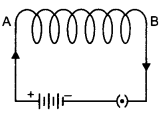(a) Solenoid.
(b) Uniform magnetic field.
(c)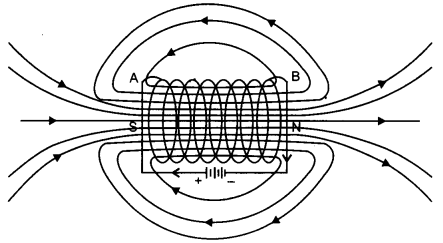(d) (i) Magnitude of current flowing throught it.
(ii) Number of turns of a circular coil.
(e) It becomes an electromagnet.

Question 28.
Explain any two situations that can cause electrical hazards in domestic circuits.  (CBSE 2013)

1. Short circuit: When the sub standard wires are used for wiring and the worn out wires comes in contact with the live wire this results in spark which further leads to major fire.
2. Overloading: When too many electrical appliances are connected at one point of the power plug and the current drawn from it is high and due to difference in the power rating the wires may melt leading to fire.

Question 29.
List in tabular form two major differences between an electric motor and a generator.   (CBSE 2013)
Electromagnet retains magnetism as long as the current flows in the enveloping coil, while a permanent magnet retains its magnetism all the time.

Question 30.
Why and when does a current carrying conductor kept in a magnetic field experience force? List the factors on which direction of this force depends?  (CBSE 2014)
In a metal conductor when the free electrons are moving in a definite direction the current is said to be induced. When this metal conductor is placed in a magnetic field the electrons experience a magnetic force which affects the conductor. Hence the current carrying conductor when placed in a magnetic field will experience a force.

The direction of the magnetic force depends on

1. direction of the current flowing through the conductor and
2. direction of the magnetic field lines.

Question 31.
How is the strength of magnetic field near a straight current-conductor
(i) related to the strength of current in the conductor?
(ii) is affected by changing the direction of flow of current in the conductor?  (CBSE 2014)
(i) The strength of magnetic field around a straight current conductor increases on increasing the strength of current in the conductor or vice versa.

(ii) The direction of magnetic field around a straight current carrying conductor gets reversed if the direction of current through that conductor is reversed.

Question 32.
What is meant by the term ‘frequency of an alternating current’? What is its value in India? Why is an alternating current considered to be advantageous over direct current for long range transmission of electric energy? (CBSE 2014)

• requency of Alternating current is equal to the number of cycles completed in one second.
• In India, frequency of AC is 50 Hz i.e., 50 cycles per second.
• An alternating current is considered to be advantageous over direct current for long range transmission of electric energy because it can be transmitted over long distances to distant places without much loss of electric power as compared to direct current.

Question 33.
State one main difference between A.C. and D.C. Why A.C. is preferred over D.C. for long range transmission of electric power? Name one source each of D.C. and A.C.  (CBSE 2014)
Difference between A.C. and D.C. The alternating current (A.C.) reverses its direction periodically whereas the direct current (D.C.) always flows in one direction.

A.C. is preferred over D.C. because it can be transmitted over long distance without much loss of energy. D.C. source: Battery A.C. source: A.C. generator.

Question 34.
State the consequences that can lead to a short circuit. Or One of the major cause of fire in office building is short circuiting. List three factors which may lead to the short circuit.   (CBSE 2014)
Short circuit can be caused due to several reasons listed below:

1. When the insulation of live wire is worn out and it comes in contact with the neutral or earth wire.
2. The water or saliva or any fluids which has ions comes in contact with the live circuit.
3. When extra current flows through a wire or if the wire is not of good quality.

Question 35.
Describe an activity to show that the magnetic field lines produced when current is passed through the circular coil. (CBSE 2015)
Take a rectangular cardboard having two holes. Insert a circular coil having large number of turns through them, normal to the plane of the cardboard.

• Connect the ends of the coil in series with a battery, a key and a rheostat.
• Sprinkle iron filings uniformly on the cardboard.
• Plug the key.
• Tap the cardboard gently a few times. Note’ the pattern of the iron filings.

Observation: The magnetic field produced is much stronger and the iron filings shows
the pattern as shown in the figure below.Question 36.
What is meant by solenoid? How does a current carrying solenoid behave? Give its main use.   (CBSE 2015)
Solenoid: Solenoid is a long coil of many turns of insulated copper wire wrapped in the shape of a cylinder.

The current carrying solenoid behave like a bar magnet.
Use: It is used to form electromagnet or permanent magnet.

Question 37.
For the current carrying solenoid as shown below, draw magnetic field lines and giving reason explain that out of the three points A, B and C at which point the field strength is maximum and at which point it is minimum.   (CBSE 2015)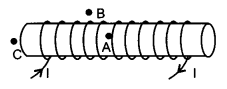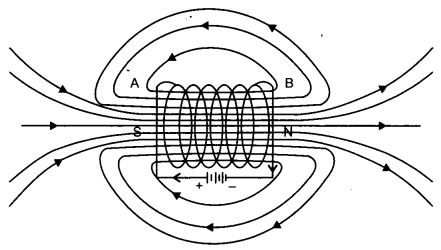The magnetic field line is maximum at point A and it is minimum at point B, C lies at the pole the field is less than A.

Question 38.
With the help of a diagram of experimental setup describe an activity to show that the force acting on a current carrying conductor placed in a magnetic field increases with increase in field strength.  (CBSE 2015)
Aim: To show that force acting on a current carrying conductor placed in a magnetic field increases with the field strength.

Apparatus Required: Aluminium rod, stand horse shoe magnet of different intensity, cell, key and connecting wires.Procedure:

• Take a small aluminium rod AB. Using two connecting wires suspend it horizontally from a stand, as shown in the figure.
• Place a strong horse-shoe magnet in such a way that the rod lies between the two poles with the magnetic field directed upwards. For this put the north pole of the magnet vertically below and south pole vertically above the aluminium rod.
• Connect the aluminium rod in series with a battery, a key and a rheostat.
• Now pass the current through the aluminium rod from end B to end A.

Observation:

• It is observed that the rod is displaced towards the left. The rod gets displaced.
• Reverse the direction of current flowing through the rod and observe the direction of its displacement. It is now towards the right.

The rod gets displaced due to the force exerted on the current carrying aluminium rod when it is placed in a magnetic field.A current-carrying rod AB, experiences a force perpendicular to its length and the magnetic field.

Now change the strength of the horseshoe magnet and place the other one with higher strength.

Observation: The rod moves faster each time as the strength of the horseshoe magnet is increased.
This shows that as the magnetic field strength is increased the rod experiences greater force and moves faster.

Conclusion: F = ma, the force acting on a current carrying conductor placed in the magnetic field increases with the increase in the strength of the field.

Question 39.
What is an electromagnet? Draw a circuit diagram to show how a soft iron piece can be transformed into an electromagnet.   (CBSE 2008)
When a material is placed inside a coil carrying current (may be solenoid) it will get magnetised. A bunch of nails or an iron rod placed along the axis of the coil can be magnetised by the current allowed to pass through the coil.Once the current is put-off the magnetic field will also be lost. Such magnets are called electromagnets.

Question 40.
What are magnetic field lines? Justify the following statements
(a) Two magnetic field lines never intersect each other.
(b) Magnetic field lines are closed curves.   (CBSE 2015)
Magnetic field lines: The region around the magnet in which the force of attraction
and repulsion is produced by the magnet is called magnetic field lines.

(a) This is because at the point of the intersection the compass needle would point in two directions which is not possible.
(b) The field lines emerge from North and merges to the South pole outside the magnet forming the closed curves.

### Magnetic Effects of Electric Current Class 10 Important Questions Long Answer Type

Question 1.
Describe an activity to draw a magnetic field line outside a bar magnet from one pole to another pole.   (CBSE 2008)
To draw magnetic field due to a bar magnet.
Apparatus required: Magnet, magnetic compass needle, white sheet, board pencil.

Procedure:1. Fix the white sheet on the board kept on a horizontal table surface.
2. Place the magnetic needle at its middle and draw a line connecting the ends of pointer using a lead pencil, parallel to the length of the board/paper.
3. Place the magnet such that the line divides the magnet equally into two parts and mark its boundary. Let the magnet’s North be on the side of the North of earth.
4. Place the compass close to the North pole and fix the needle positions.
5. Shift the needle such that the second point is one of the position of the needle and mark the other needle’s position.
6. Repeat the step 5 till you reach the south pole of the magnet and join all the
points to make a curve.
7. Repeat the steps 4 to 6 for either side of the magnet.
8. Draw same lines away to the poles of the magnet near the edges/poles.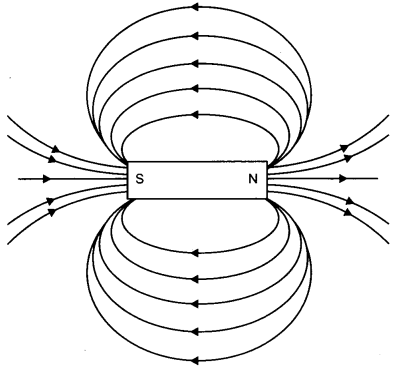Question 2.
Draw a diagram to show the magnetic field lines around a bar magnet. List any two properties of magnetic field lines.   (AI 2008/2011)Properties:

1. Tangent at any points gives the direction of magnetic field.
2. No two lines can intersect.

Question 3.
(a) Describe an activity to demonstrate the pattern of magnetic field lines around a straight conductor carrying current.
(b) State the rule to find the direction of magnetic field associated with a current carrying conductor.
(c) Two room heaters are marked 220 V, 500 W and 200 V, 800 W respectively. If the heaters are connected in parallel to 220 V mains supply, calculate
(i) the current drawn by each heater.
(ii) the resistance of each heater.
(iii) total electric energy consumed in commercial units if they operate simultaneously for 2 hours.

(Or)

(a) State Fleming’s Right Hand Rule.
(b) (i) Name the electric device that converts mechanical energy into electrical
energy.
(ii) Write the principle involved in this device.
(c) An electric geyser of 2 kW rating is operated in a domestic circuit operating on
220 V main supply that has a fuse of current rating of 5 A. What will be outcome? Explain.   (AI 2008/2011)
(a) Aim: To study the magnetic field due to a straight conductor carrying current.
Apparatus Required: A thick conducting wire, battery, rheostat, magnetic needle, ammeter (0-5 A), key, a cardboard a stand to hold the wire, iron filings and sprinkler of iron filings.

Procedure:

• Attach the thick wire through a hole at the middle of the cardboard and clamp it in a stand.
• Attach the ends of wires through a key, variable resistor and an ammeter on either side to a battery and hold the same vertically perpendicular to the board.
• Spread the iron fillings uniformly on the cardboard and place the magnetic needle on the board.
• Close the key and tap the cardboard slightly and observe the orientation of iron filings and the magnetic field.
• Move the needle slightly away and observe the change.
• Change the rheostat positioning to vary the current (from ammeter) and observe the change in the needle.
• Connect the battery in the reverse and observe the change in the needle’s orientation.

Observations:

• Just on closing the key the iron filings aligned in an order.
• As the current was sent in the downward direction through the wire the North pole of the field produced was pointing along the tangent in a clockwise direction.
• Movement of needle slightly away caused a reduction in its deflection.
• As the current was altered, there was a change in the deflection of needle.
• As the current was reversed, the needle reversed its direction.

Conclusions:

• Current carrying conductor is a source of magnetic field.
• Direction of current will determine the direction of magnetic field-reversal of current reverses magnetic field.
• Magnetic field B produced depends on the distance r of needle from the wire B ∝ $$\frac{1}{r}$$, i.e., B reduces with increase in distance r.
• Variation of magnitude of current causes changes in the strength of the field B can be found to increase with increase in the current.

(b) Right Hand Thumb Rule: Hold the wire carrying current in your right hand, such that the thumb indicates the direction of current, then the folded fingers will indicate the direction of magnetic field (lines) surrounding the wire.

(c) (i) Current drawn by 500 W heater I1 = P1/V = 500/220 = 2.27 A
Current drawn by 800 W heater = I2 = P2/V = 800/220 = 3.64 A
(ii) Resistance of 500 W heater = R1 = $$\frac{220}{I_{1}}$$ = 97
(iii) Energy consumed in 2 hours = P1t + P2t
= (500 x 2) + (800 x 2) = 2600 Wh
= 2.6 kwh or 2.6 units

(or)

(a) Fleming’s Right Hand Rule: Stretch the first three fingers of the right hand mutually perpendicular to each other such that the fore-finger gives the direction of magnetic field and the thumb points the direction of the motion of a conductor than the middle finger will give the direction of induced current.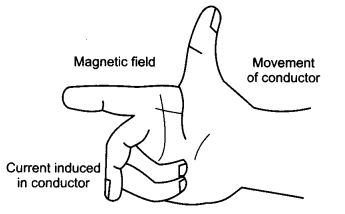(b) (i) AC generator
(ii) Electric generators works on the principle of electromagnetic induction. It converts the mechanical energy available into electrical energy when a coil is rotated in a magnetic field, when there will be induced current flowing in it. The direction of induced current can be found using the Fleming’s right hand rule.

(c) When 2 kW geyser is run on 220 Volt supply the current drawn = I = P/V = $$\frac{2 \times 1000}{220}=\frac{200}{22}$$ > 5A. The fuse with a current rating 5 A will fuse and the geyser cannot work.

Question 4.
(a) What is a magnetic field? How can the direction of magnetic field lines at a place be determined?
(b) State the rule for direction of the magnetic field produced around a current carrying conductor. Draw a sketch of the pattern of field lines due to a current flowing through a straight conductor.
(Or)
(a) What is a solenoid? Draw a sketch of the pattern of field lines of the magnetic field through and around a current carrying solenoid.
(b) Consider a circular loop of wire lying in the plane of the table. Let the current pass through the loop clockwise. Apply the right hand rule to find out the direction of the magnetic field inside and outside the loop.
(a) Magnetic Field: The region or space around a magnet where magnetic force can be experienced by other magnetic substance is called magnetic field. It is measured in tesla or weber/m2.

(b) Right Hand Thumb Rule: Hold the wire carrying current in your right hand, such that the thumb indicates the direction of current, then the folded fingers will indicate the presence of magnetic field lines surrounding the wire.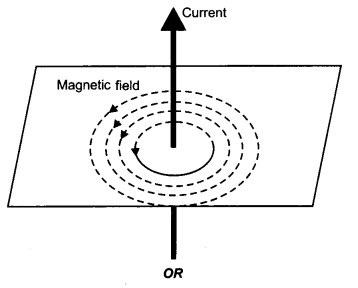(a) Solenoid: A coil of large number of turns closely wound on a hollow cylinder of insulated material or otherwise is called a solenoid. The end of solenoid having clockwise current will act as South pole (field enters into), while the other end having anticlockwise current will act as North pole (field comes out).

Thus a solenoid acts as a normal magnet the field diverges at the edges of the solenoid and so there is reduction in its strength. However inside the solenoid the lines are parallel indicating a uniform magnetic field.

The strength of the uniform magnetic field inside depends on (i) Number of turns per unit length of the solenoid and (ii) the current passing through it.(b) If we hold this loop such that the thumb points to the direction of current then the curly finger will get into the loop from the top and come out of the loop at its bottom. This make the force of the loop above the table as “South pole”. Indicated as “S”.
The field lines outside and inside it can be shown easily as below.Magnetic field due to a current carrying circular loop:
The field created due to a circular coil is given in figure.
The direction of fields is perpendicular to the plane of the coil and direct inwards. For the clockwise current and for anticlockwise current it will be following anticlockwise. The field lines in circular coil have the following characters.

• Circular near the wire.
• Straight at the centre.
• Field is perpendicular to the plane of the coil.
• For large areas, the field at the centre is uniform.
• Field lines will enter into one plane and leave the other. (Enter on the side with clockwise current).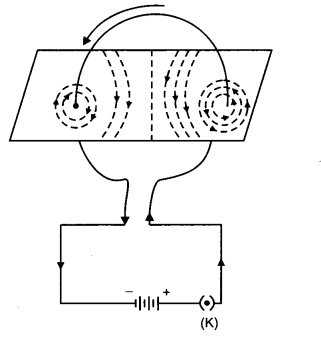Question 5.
(a) Draw a schematic labelled diagram of a domestic wiring circuit which includes
(i) a main fuse
(ii) a power meter
(iii) one light point
(iv) a power output socket
(b) In this circuit on which wire of the circuit is the mains on/off switch connected?(b) Mains ON/OFF switch is connected in the neutral wire either at point A or B.

Question 6.
(a) An electric bulb is rated as 50 W, 220 V, calculate the energy consumed by the bulbs in 20 minutes. Express your answer in commercial units of electric energy.
(c) Why is it essential to earth electrical appliances having metallic body?   (CBSE 2008)
(a) P = 50 W
t = 20 minutes
V = 220 V
t = ?
∴ E = P × t
P = 50 W = $$\frac{50}{1000}$$kW = 0.05 kW
t = 20 minutes = $$\frac{20}{60}$$h = $$\frac{1}{3}$$
E = P × t = 0.05 x $$\frac{1}{3}$$ = 0.016 kWh.

(b)

1. Large amount of current is drawn from single point.

Short Circuiting:

1. Two wires come in contact with each other (naked wires) like live wire and earth or neutral wire.

(c) Metallic bodies are good conductors of electricity, when we plug in these appliances, there is a tendency of flow of electric current through the appliance to a person who touch these appliances and can cause electrocution. To avoid this metallic bodies are earthed.

Question 7.
(a) Describe an activity to demonstrate the pattern of magnetic field lines around a straight conductor carrying current.
(b) State the rule to find the direction of magnetic field associated with a current carrying conductor.
(c) What is the shape of a current-carrying conductor whose magnetic field pattern resembles that of a bar-magnet?   (CBSE 2011)
(a) Magnetic Field: The region or space around a magnet where magnetic force can be experienced by other magnetic substance is called magnetic field. It is measured in tesla or weber/m2.

(b) Right Hand Thumb Rule: Hold the wire carrying current in your right hand, such that the thumb indicates the direction of current, then the folded fingers will indicate the presence of magnetic field lines surrounding the wire.(c) Solenoid:
A coil of large number of turns closely wound on a hollow cylinder of insulated material or otherwise is called a solenoid. The end of solenoid having clockwise current will act as South pole (field enters into), while the other end having anticlockwise current will act as North pole (field comes out).

Thus a solenoid acts as a normal magnet the field diverges at the edges of the solenoid and so there is reduction in its strength. However inside the solenoid the lines are parallel indicating a uniform magnetic field.

The strength of the uniform magnetic field inside depends on (i) Number of turns per unit length of the solenoid and (ii) the current passing through it.Question 8.
(a) Draw a diagram to represent a uniform magnetic field in a given region.
(b) List two properties of magnetic field lines.   (CBSE 2011)
(a) Equidistant parallel line indicates a uniform magnetic field in a given region.Question 9.
Two coils C1 and C2 are wrapped around a non conducting cylinder. Coil C1 is connected to a battery and key and C2 with galvanometer G. On pressing the key (K), current starts flowing in the coil C1. State your observation in the galvanometer.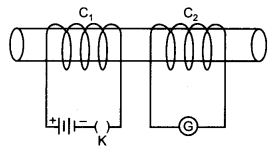(i) When key K is pressed on
(ii) When current in the coil C1 is switched off.
(iii) When the current is passed continuously through coil C1
(iv) Name and state the phenomenon responsible for the above observation. Write
the name of the rule that is used to determine the direction of current produced in the phenomena.  (CBSE 2013)
(i) When key is pressed on, the galvanometer needle deflects momentarily in one direction.
(ii) When the current in the coil C1 is switched off, the galvanometer needle deflects again momentarily but in opposite direction to that in the previous case.
(iii) When current is passed continuously through coil C1, no deflection is observed in the galvanometer.
(iv) The phenomenon responsible for the above observations is electromagnetic induction. The process, by which a changing magnetic field in a conductor induces a current in another conductor placed nearby, is called electromagnetic induction.

Fleming’s right hand rule is used to determine the direction of current produced in the phenomena of electromagnetic induction.

Question 10.
(a) Describe an activity to demonstrate the pattern of magnetic field lines around a straight conductor carrying current.
(b) State the rule to find the direction of magnetic field associated with a current carrying conductor.
(c) What is the shape of current carrying conductor whose magentic field pattern resembles that of a bar-magnet?   (CBSE 2014)

• Take a battery of 12 V a variable resistance or a rheostat, an ammeter (0-5 A), a plug key and a long straight thick copper wire.
• Insert the thick wire through the centre, normal to the plane of rectangular card­board. Take care that the cardboard is fixed and does not slide up or down.
• Connect the copper wire vertically between the points X and Y as shown in the figure, in series with the battery, plug and key.
• Sprinkle some iron filings uniformly on the cardboard.
• Keep the variable of the rheostat at a fixed position and note the current through the ammeter.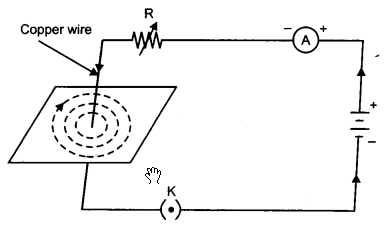A pattern of concentric circles indicating the field lines of a magnetic field around a straight conducting wire. The arrow shows the direction of the field lines.

• Close the key so that a current flows through the wire. Ensure that the copper wire placed between the points X and Y remains vertically straight.
• Gently tap the cardboard a few times. Observe the pattern of the iron filings. The iron filings align themselves showing a pattern of concentric circles around the copper wire.
• These concentric circles represent the magnetic field lines.
• With the help of a compass needle, the direction of the field lines can be observed.
• When the current through the wire is reversed the direction of magnetic field lines also gets reversed.

Question 11.
(a) Explain why there are two separate circuits one for high power rating appliances and other for low power rating appliances.
(b) A domestic circuit has 5A fuse. How many bulbs of rating 100 W, 220 V can be safely used in this circuit? Justify your answer. (CBSE 2014)
(a) The high power circuit carries high current of 15 A and is use for the electrical appliances with high rating of power. The lower circuit carries the current of 5 A and the appliances which need low current is used in this circuit.

Both these circuits are separate because the low rated appliances may get soiled if extra current flows through it and the high rated appliances will not work effectively if low current is flowing through it.

(b) I = 5A,  V = 220 V,  P = n × 100 W,   n = number of bulbs
I = P/V
Hence 5 = n × 100/220 ⇒  n = 1100/100 = 11 bulbs.

Question 12.
(a) Describe activity with labelled diagram to show that a current carrying conductor experience a force in a magnetic field.
(b) State the rule to determine the direction of force.   (CBSE 2015)